# Maharashtra Board Class 5 Maths Solutions Chapter 3 Addition and Subtraction Problem Set 10

Balbharti Maharashtra Board Class 5 Maths Solutions Chapter 3 Addition and Subtraction Problem Set 10 Textbook Exercise Important Questions and Answers.

## Maharashtra State Board Class 5 Maths Solutions Chapter 3 Addition and Subtraction Problem Set 10

Question 1.
Subtract the following:

(1) 64293

28547
________
________
Solution:
6 4 2 9 3

2 8 5 4 7
3 5 7 4 6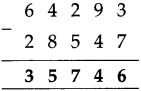(2) 37058

23469
________
________
Solution:
3 7 0 5 8

2 3 4 6 9
1 3 5 8 9(3) 71540

58628
________
________
Solution:
7 1 5 4 0

5 8 6 2 8
1 2 9 1 2(4) 50432

48647
________
________
Solution:
5 0 4 3 2

4 8 6 4 7
0 1 7 8 5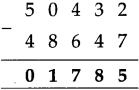Subtraction of six and seven-digit numbers

You have learnt to carry out subtractions of five-digit numbers. Using the same method, we can do subtractions of numbers with more than five digits. Study the following examples.Subtract :
Example (1) 65,07,843 – 9,25,586Example (2) 34,61,058 – 27,04,579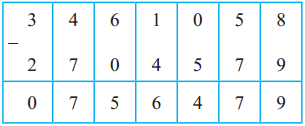As shown in the above example, learn to subtract by keeping the borrowed numbers in your mind without writing them down.

Subtraction by another method Before subtracting one number from another, if we add 10 or 100 to both of them, the difference remains the same. Let us use this fact.

Example : Subtract : 724 – 376
As we cannot subtract 6 from 4, we shall add a ten to both the numbers. For the upper number, we untie one tens. We add those ten units to 4 units to get 14 units.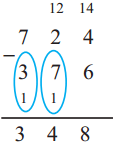We write the tens added to the lower number below it, in the tens place.

We subtract 6 units from 14.

Now, we cannot subtract ( 7 + 1) i.e. 8 tens from 2 tens. So, we add one hundred to both the numbers. For the upper number, we untie the hundred and add the ten tens to 2 tens. To add the hundred to the lower number, we write it below, in the hundreds place. 12 tens minus 8 tens is 4 tens. And 7 hundreds minus (3 + 1) i.e. 4 hundreds is 3 hundreds. Hence, the difference is 348.

Example (1)Example (2)Question 1.
Subtract the following:

(1) 81,345 – 35,667
Solution:
8 1 3 4 5

3 5 6 6 7
4 5 6 7 8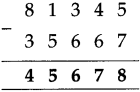(2) 64,723 – 52,378
Solution:
6 4 7 2 3

5 2 3 7 8